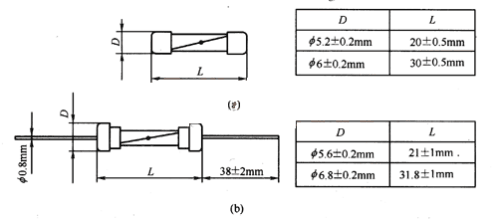﻿ Can Fuse Resistors Be Used Instead of Current Fuse?_

China Thermal Protector Manufacturer

# Can Fuse Resistors Be Used Instead of Current Fuse?

Fuse resistance and current fuse in the circuit's role is different, Fuse resistance is a low resistance value of the current limiting protection devices, From overload protection and fuse protection! Fuse is a zero-resistance short-circuit protection element, The former is a transitional reaction, The latter is an instant reaction!Circuit Design Current fuse + resistor can replace the fuse resistance, But this design will cause the cost to increase.
Yaxun electronic sub-fuse fuse resistance and current exchange characteristics:

1, the basic characteristics of the fuse resistance:
Insulation resistance wire wound type, carbon film type, metal film type, oxide film type and chemical deposition film type. One wire wound type of insurance resistance is low, Applicable to high current conditions. Four kinds of film-type fuse resistance is not the main products, The matrix is low melting point boron lead silicate, And contains a small amount of potassium, sodium ions, the formation of a more relaxed structure. It is extremely easy to fail after heating, to achieve safety and protection. Is the main technical indicators of film-type fusing resistor.

Fuse resistance drop power curve shown in Figure 1:Note: The rated power is 70 ℃ in the temperature of the maximum continuous work, more than 70 ℃ by the curve down power consumption.

Fuse and resistance characteristics of the fuse resistance is the most important indicators, In the circuit diagram plays a dual role of the fuse and resistance, Mainly used in the power circuit output and the secondary power output circuit. They are generally low resistance (several ohms to tens of ohms), Small power (1/8 ~ 1W) for many, Is the actual power consumption of the circuit several times the rated power, After the continuous load operation for a certain time, In the provisions of the ambient temperature range to ensure that the resistance fuse, Protects other components in the circuit from damage.

2, the basic characteristics of current fuse:
The resistance of the fuse is not important in the entire circuit. As the amperage is less than 1 fuse resistance of only a few ohms, So in the low-voltage circuit with a fuse should be considered when the problem. Most of the fuse is a positive temperature coefficient of the material, Therefore, there are cold resistance and thermal resistance of the points.

Current fuse and heat coefficient:
When the current flows through it, it will heat, The amount of heat is also increasing with time. The magnitude of the current and the resistance determines the rate at which heat is generated,
The construction of the fuse and the condition of its installation determine the rate of heat dissipation, If the rate of heat generation is less than the rate of heat dissipation, the fuse is not blown. If the speed of heat generation is equal to the rate of heat dissipation, In a very long time it will not blow. If the speed of heat generation is greater than the rate of heat dissipation, Then the heat will be more and more. And because it has a certain heat and quality, The increase in heat on the performance of the temperature rise, The fuse blows when the temperature rises above the melting point of the fuse.

The current fuse to reduce the power curve melting temperature Figure 2:

It can be seen from the curve of the fuse fuse material has a lower melting temperature, Relatively sensitive to the temperature of the relatively large reduction rate.

3, the current fuse and fuse interchangeability characteristics:
Once the fuse resistance burned out, Should first identify the burning fuse resistance of the original solid, will not allow blind replacement, More can not use ordinary resistance substitution. If there is no fuse of the same specification, Emergency replacement can be carried out as follows.
1. Resistance and fuse in series with the alternative, A resistor and a fuse (or fuse) in series instead, Resistance of the resistance, power and fuse resistance specifications. For example, the original fuse resistance specifications for the 10Ω, 2W, The resistance can be selected 10Ω / 2W, The rated current I of the fuse is given by the square of the formula I R = 56% P where R is the resistance value (Ω) P is the rated power (w). In this case, the rated current should be 0. 3A.
2. Directly with the fuse substitution
Some small resistance of the fuse resistance is damaged, Can be directly used with a fuse. This method is suitable for less than 1Ω fuse resistance, Fuse fuse current value according to the above formula from the original insurance resistance calculation.

﻿
﻿